Courses

# Olympiad Test: Surds And Indices

## 20 Questions MCQ Test Mathematics Olympiad Class 7 | Olympiad Test: Surds And Indices

Description
This mock test of Olympiad Test: Surds And Indices for Class 7 helps you for every Class 7 entrance exam. This contains 20 Multiple Choice Questions for Class 7 Olympiad Test: Surds And Indices (mcq) to study with solutions a complete question bank. The solved questions answers in this Olympiad Test: Surds And Indices quiz give you a good mix of easy questions and tough questions. Class 7 students definitely take this Olympiad Test: Surds And Indices exercise for a better result in the exam. You can find other Olympiad Test: Surds And Indices extra questions, long questions & short questions for Class 7 on EduRev as well by searching above.
QUESTION: 1

### ​If x = 3 + 2√2 , then the value of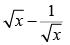is:

Solution:

Here,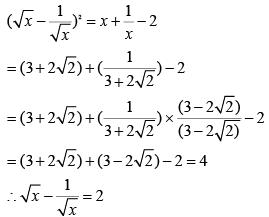QUESTION: 2

###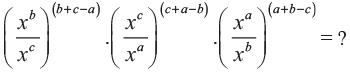Solution:

Given Expression
= x(b – c)(b + c – a). x(c – a)(c + a – b)
. x(a – b)(a + b – c)
= x(b – c)(b + c) – a(b – c) . x(c - a)(c + a) – b(c – a)
. x(a - b)(a + b) - c(a - b)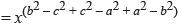. x–a(b – c) – b(c – a) – c(a – b)
= (x0 × x0)
= (1 × 1) = 1

QUESTION: 3

### If m and n are whole numbers such that mn = 121, the value of (m – 1)n + 1 is:

Solution:

We know that 112 = 121 = mn (given)
Hence, putting m = 11 and n = 2, in
(m – 1)n + 1 = (11 – 1)(2 + 1) = 103 = 1000

QUESTION: 4

(256)0.16 × (256)0.09 = ?

Solution:

(256)0.16 × (256)0.09 = (256)(0.16 + 0.09)
= (256)0.25
= (256)(25/100)
= (256)(1/4)
= (44)(1/4)
= 44(1/4)
= 41
= 4

QUESTION: 5

The value of [(10)150 ÷ (10)146] is

Solution:

(10)150 ÷ (10)146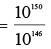= 10150 – 146
= 104
= 10000

QUESTION: 6

If (25)7.5 × (5)2.5 ÷ (125)1.5 = 5x then x = ?

Solution:

Given (25)7.5 × (5)2.5 ÷ (125)1.5 = 5x
Then, (52)7.5 × (5)2.5 ÷ (53)1.5 = 5x
⇒ 5(2 × 7.5) × 52.5 ÷ 5(3 × 1.5) = 5x
⇒ 515 × 52.5 ÷ 54.5 = 5x
⇒ 5x = 5(15 + 2.5 – 4.5)
⇒ 5x = 513
⇒ x = 13

QUESTION: 7

(0.04)–1.5 = ?

Solution:

(0.04) – 1.5 = (4/100) –1.5 = (1/25) –3/2
= (25)(3/2)
= (52)(3/2)
= (5)2 × (3/2)
= 53
= 125

QUESTION: 8

If 3(x – y) = 27 and 3(x + y) = 243, then x is equal to:

Solution:

3x – y = 27 = 33 ⇒ x – y = 3 ....(i)
3x + y = 243 = 35 ⇒ x + y = 5 .... (ii)
On solving (i) and (ii), we get x = 4.

QUESTION: 9

If 5a = 3125, then the value of 5(a – 3) is:

Solution:

5a = 3125 ⇔ 5a = 55
⇒ a = 5
∴ 5(a – 3) = 5(5 – 3) = 52 = 25

QUESTION: 10

Given that 100.48 = x, 100.70 = y and xz = y2, then the value of z is close to:

Solution:

xz = y2
⇒ 10(0.48z) = 10(2 × 0.70) = 101.40
⇒ 0.48z = 1.40
⇒ z =140/48 = 35/12 = 2.9 (approx.)

QUESTION: 11

(17)3.5 × (17)? = 178

Solution:

Let (17)3.5 × (17)x = 178
Then, (17)3.5 + x = 178
∴ 3.5 + x = 8
⇒ x = (8 – 3.5)
⇒ x = 4.5

QUESTION: 12

Find the value of √300.

Solution: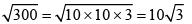= 10 × 1.732 = 17.32

QUESTION: 13

Evaluate: √75 + √147 =?

Solution: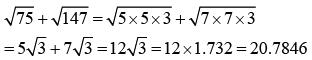QUESTION: 14

Find the value of: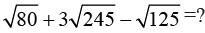Solution: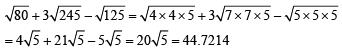QUESTION: 15

Find √242 ÷ √72 .

Solution: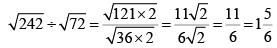QUESTION: 16

(18a8b6) ÷ (3a2b2) simplifies to

Solution:

(18a8b6) ÷ (3a2b2) = 18/3 × a8 – 2 × b6 – 2
= 6a6b4

QUESTION: 17

Replace question mark with the suitable answer:
56 - 45- √? = √36

Solution:

56 - 45 -√? = √36
⇒ 11 - √36 =√?
⇒ 11 - 6 =√?
∴ ? = 25

QUESTION: 18

Replace question mark with the suitable answer: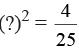Solution: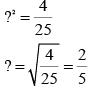QUESTION: 19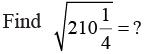Solution: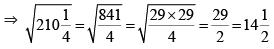QUESTION: 20

What is the value of (0.003)3 ?

Solution:

(0.003)3 = 0.003 × 0.003 × 0.003
= 0.000000027